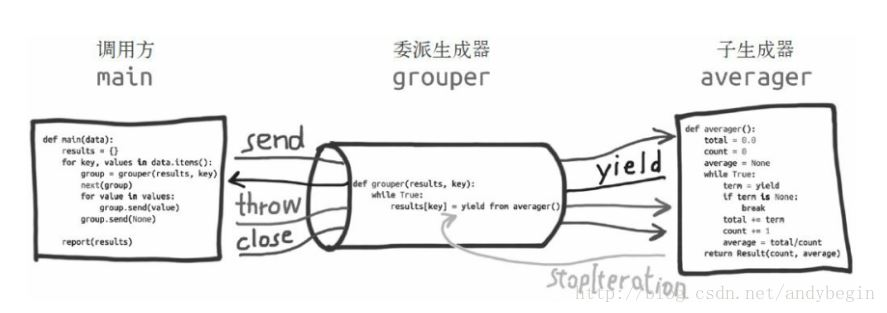# 协程的详尽用法使用和例子

.send(datum) 方法，而不是next(…) 函数。

1. 委派生成器在 yield from
表达式处暂停时，调用方可以直接把数据发给子生成器。
2. 子生成器再把产出的值发给调用方。
3. 子生成器返回之后，解释器会抛出 StopIteration
异常，并把返回值附加到异常对象上，此时委派生成器会恢复。

==yield from 结构会在内部自动捕获 StopIteration 异常==。对 yield from

yield from 表达式的值。

datum = yield），可以产出值，也可以不产出 —— 如果 yield

==最先调用 next(sc)

yield 表达式，准备好作为活跃的协程使用）。

``def gen(): for c in 'AB': yield cprint(list(gen()))def gen_new(): yield from 'AB'print(list(gen_new()))``
``import collectionsimport queueimport random# time 字段是事件发生时的仿真时间，# proc 字段是出租车进程实例的编号，# action 字段是描述活动的字符串。Event = collections.namedtuple('Event', 'time proc action')def taxi_process(proc_num, trips_num, start_time=0): """ 每次改变状态时创建事件，把控制权让给仿真器 :param proc_num: :param trips_num: :param start_time: :return: """ time = yield Event(start_time, proc_num, 'leave garage') for i in range(trips_num): time = yield Event(time, proc_num, 'pick up people') time = yield Event(time, proc_num, 'drop off people') yield Event(time, proc_num, 'go home')class SimulateTaxi(object): """ 模拟出租车控制台 """ def __init__(self, proc_map): # 保存排定事件的 PriorityQueue 对象， # 如果进来的是tuple类型，则默认使用tuple做排序 self.events = queue.PriorityQueue() # procs_map 参数是一个字典,使用dict构建本地副本 self.procs = dict(proc_map) def run(self, end_time): """ 排定并显示事件，直到时间结束 :param end_time: :return: """ for _, taxi_gen in self.procs.items(): leave_evt = next(taxi_gen) self.events.put(leave_evt) # 仿真系统的主循环 simulate_time = 0 while simulate_time < end_time: if self.events.empty(): print('*** end of events ***') break # 第一个事件的发生 current_evt = self.events.get() simulate_time, proc_num, action = current_evt print('taxi:', proc_num, ', at time:', simulate_time, ', ', action) # 准备下个事件的发生 proc_gen = self.procs[proc_num] next_simulate_time = simulate_time + self.compute_duration() try: next_evt = proc_gen.send(next_simulate_time) except StopIteration: del self.procs[proc_num] else: self.events.put(next_evt) else: msg = '*** end of simulation time: {} events pending ***' print(msg.format(self.events.qsize())) @staticmethod def compute_duration(): """ 随机产生下个事件发生的时间 :return: """ duration_time = random.randint(1, 20) return duration_time# 生成3个出租车，现在全部都没有离开garagetaxis = {i: taxi_process(i, (i + 1) * 2, i * 5) for i in range(3)}# 模拟运行st = SimulateTaxi(taxis)st.run(100)``

``def simple_coroutine(): print('-> start') x = yield print('-> recived', x)sc = simple_coroutine()next(sc)sc.send('zhexiao')``

yield 表达式，而产出的值会成为调用
generator.throw方法得到的返回值。如果生成器没有处理抛出的异常，异常会向上冒泡，传到调用方的上下文中。

yield from基本用法

==终止协程的一种方式：发送某个哨符值，让协程退出。内置的 None 和Ellipsis

1. 子生成器产出的值都直接传给委派生成器的调用方（即客户端代码）。
2. 使用 send()
方法发给委派生成器的值都直接传给子生成器。如果发送的值是None，那么会调用子生成器的
next() 方法。如果发送的值不是 None，那么会调用子生成器的 send()
方法。如果调用的方法抛出 StopIteration
异常，那么委派生成器恢复运行。任何其他异常都会向上冒泡，传给委派生成器。
3. 生成器退出时，生成器（或子生成器）中的 return expr 表达式会触发
StopIteration(expr) 异常抛出。
4. yield from 表达式的值是子生成器终止时传给 StopIteration
异常的第一个参数。
5. 云顶娱乐棋牌游戏 ，传入委派生成器的异常，除了 GeneratorExit 之外都传给子生成器的
throw() 方法。如果调用 throw() 方法时抛出 StopIteration
异常，委派生成器恢复运行。 StopIteration
之外的异常会向上冒泡，传给委派生成器。
6. 如果把 GeneratorExit 异常传入委派生成器，或者在委派生成器上调用
close() 方法，那么在子生成器上调用 close()
方法，如果它有的话。如果调用close()方法导致异常抛出，那么异常会向上冒泡，传给委派生成器；否则，委派生成器抛出GeneratorExit
异常。

yield from 专门的术语

#### 您可能感兴趣的文章:

• Python中协程用法代码详解
• 浅析python协程相关概念
• Python协程的用法和例子详解
• python 生成器协程运算实例
• python简单线程和协程学习心得(分享)
• python并发编程之多进程、多线程、异步和协程详解
• python线程、进程和协程详解
• 深入浅析python中的多进程、多线程、协程
• 简述Python中的进程、线程、协程
• python协程用法实例分析
1. 委派生成器：包含 yield from 表达式的生成器函数。
2. 子生成器：从 yield from 中 部分获取的生成器。
``from collections import namedtupleResClass = namedtuple('Res', 'count average')# 子生成器def averager(): total = 0.0 count = 0 average = None while True: term = yield if term is None: break total += term count += 1 average = total / count return ResClass(count, average)# 委派生成器def grouper(storages, key): while True: # 获取averager()返回的值 storages[key] = yield from averager()# 客户端代码def client(): process_data = { 'boys_2': [39.0, 40.8, 43.2, 40.8, 43.1, 38.6, 41.4, 40.6, 36.3], 'boys_1': [1.38, 1.5, 1.32, 1.25, 1.37, 1.48, 1.25, 1.49, 1.46] } storages = {} for k, v in process_data.items(): # 获得协程 coroutine = grouper(storages, k) # 预激协程 next(coroutine) # 发送数据到协程 for dt in v: coroutine.send(dt) # 终止协程 coroutine.send(None) print(storages)# runclient()``

I/O，以及其他事件驱动型编程形式或协作式多任务。协程是 asyncio

``import collections# time 字段是事件发生时的仿真时间，# proc 字段是出租车进程实例的编号，# action 字段是描述活动的字符串。Event = collections.namedtuple('Event', 'time proc action')def taxi_process(proc_num, trips_num, start_time=0): """ 每次改变状态时创建事件，把控制权让给仿真器 :param proc_num: :param trips_num: :param start_time: :return: """ time = yield Event(start_time, proc_num, 'leave garage') for i in range(trips_num): time = yield Event(time, proc_num, 'pick up people') time = yield Event(time, proc_num, 'drop off people') yield Event(time, proc_num, 'go home')# runt1 = taxi_process(1, 1)a = next(t1) print(a) # Event(time=0, proc=1, action='leave garage')b = t1.send(a.time + 6)print(b) # Event(time=6, proc=1, action='pick up people')c = t1.send(b.time + 12)print(c) # Event(time=18, proc=1, action='drop off people')d = t1.send(c.time + 1)print(d) # Event(time=19, proc=1, action='go home')``
1. 外层 for 循环每次迭代会新建一个 grouper 实例，赋值给 coroutine 变量；
grouper 是委派生成器。
2. 调用 next(coroutine)，预激委派生成器 grouper，此时进入 while True
循环，调用子生成器 averager 后，在 yield from 表达式处暂停。
3. 内层 for 循环调用 coroutine.send(value)，直接把值传给子生成器
averager。同时，当前的 grouper 实例（coroutine）在 yield from
表达式处暂停。
4. 内层循环结束后， grouper 实例依旧在 yield from 表达式处暂停，因此，
grouper函数定义体中为 results[key] 赋值的语句还没有执行。
5. coroutine.send(None) 终止 averager 子生成器，子生成器抛出
StopIteration 异常并将返回的数据包含在异常对象的value中，yield from
可以直接抓取 StopItration 异常并将异常对象的 value 赋值给 results[key]

1. 协程使用生成器函数定义：定义体中有 yield 关键字。
2. yield 在表达式中使用；如果协程只需从客户那里接收数据，那么产出的值是
None —— 这个值是隐式指定的，因为 yield 关键字右边没有表达式。
3. 首先要调用 next(…) 函数，因为生成器还没启动，没在 yield
语句处暂停，所以一开始无法发送数据。
4. 调用send方法，把值传给 yield
的变量，然后协程恢复，继续执行下面的代码，直到运行到下一个 yield
表达式，或者终止。

==yield from x 表达式对 x 对象所做的第一件事是，调用
iter(x)，从中获取迭代器，因此， x 可以是任何可迭代的对象，这只是 yield
from 最基础的用法==。

1. GEN_CREATED：等待开始执行
2. GEN_RUNNING：解释器正在执行
3. GEN_SUSPENED：在yield表达式处暂停
4. GEN_CLOSED：执行结束

==yield 关键字甚至还可以不接收或传出数据。不管数据如何流动， yield

generator.close()

``def averager(): total = 0.0 count = 0 avg = None while True: num = yield avg total += num count += 1 avg = total/count# runag = averager()# 预激协程print(next(ag)) # Noneprint(ag.send(10)) # 10print(ag.send(20)) # 15``
1. 调用 next(ag) 函数后，协程会向前执行到 yield 表达式，产出 average
变量的初始值——None。
2. 此时，协程在 yield 表达式处暂停。
3. 使用 send() 激活协程，把发送的值赋给 num，并计算出 avg 的值。
4. 使用 print 打印出 yield 返回的数据。

``generator.throw(exc_type[, exc_value[, traceback]])``

==注意：send方法只有当协程处于 GEN_SUSPENDED

==yield from

yield from高级用法

==在生成器 gen 中使用 yield from subgen() 时， subgen

subgen。与此同时， gen 会阻塞，等待 subgen 终止==。yield from获取协程的返回值

``import inspectdef simple_coroutine(a): print('-> start') b = yield a print('-> recived', a, b) c = yield a + b print('-> recived', a, b, c)# run sc = simple_coroutine(5)next(sc)sc.send(6) # 5, 6sc.send(7) # 5, 6, 7``

``class DemoException(Exception): """ custom exception """def handle_exception(): print('-> start') while True: try: x = yield except DemoException: print('-> run demo exception') else: print('-> recived x:', x) raise RuntimeError('this line should never run')he = handle_exception()next(he)he.send(10) # recived x: 10he.send(20) # recived x: 20he.throw(DemoException) # run demo exceptionhe.send(40) # recived x: 40he.close()``

yield from的意义

``he.throw(Exception) # run demo exception``

Comment ()